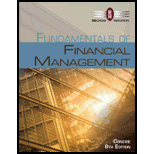Chapter 9, Problem 2PFundamentals of Financial Manageme...

8th Edition
Eugene F. Brigham + 1 other
ISBN: 9781285065137

Solutions

Chapter
SectionFundamentals of Financial Manageme...

8th Edition
Eugene F. Brigham + 1 other
ISBN: 9781285065137
Textbook Problem

CONSTANT GROWTH VALUATION Thomas Brothers is expected to pay a $0.50 per share dividend at the end of the year (that is, D1.=$0.50). The dividend is expected to grow at a constant rate of 7% a year. The required rate of return on the stock, rs is 15%. What is the stock's current value per share?

Summary Introduction

To compute: The current value per share for Company T’s stock.

Dividends per Share:

The periodic rewards received by the stockholders for their investment in a company are known as dividends. It is also measure on the basis of per share. Per share calculation of dividends is called dividends per share.

Explanation

Given,

Dividend at year 1 is $0.50. Growth rate is 7%. Required rate of return is 15%. Formula to compute current value per share, Value per Share=Dividend at Year1Required Rate of ReturnGrowth Rate Substitute$0

Still sussing out bartleby?

Check out a sample textbook solution.

See a sample solution

The Solution to Your Study Problems

Bartleby provides explanations to thousands of textbook problems written by our experts, many with advanced degrees!

Get Started

Find more solutions based on key concepts